Algebra 1 8-5 Complete Lesson: Factoring x² + bx + c
starstarstarstarstarstarstarstarstarstar
4.5 (1 rating)
by Matthew Richardson
| 28 Questions
Note from the author:
A complete formative lesson with embedded slideshow, mini lecture screencasts, checks for understanding, practice items, mixed review, and reflection. I create these assignments to supplement each lesson of Pearson's Common Core Edition Algebra 1, Algebra 2, and Geometry courses. See also mathquest.net and twitter.com/mathquestEDU.The outlined content above was added from outside of Formative.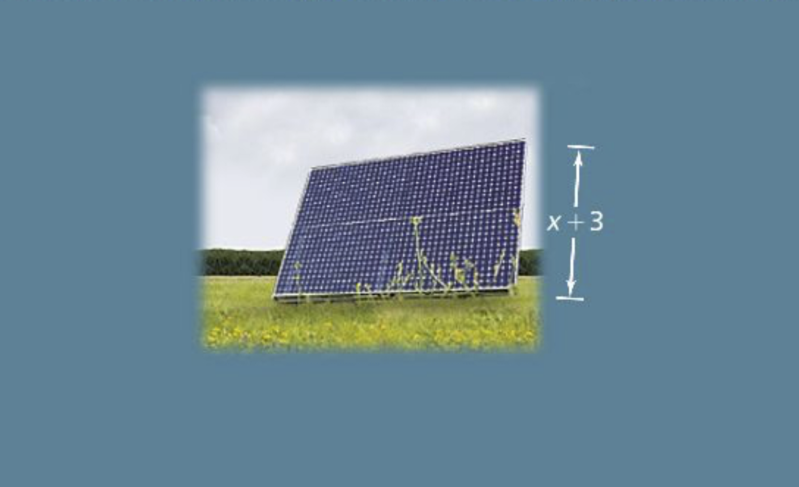1
1
10 pts
Solve It! The area of a rectangular solar panel is given by the trinomial below.
The height of the solar panel is given by the binomial below.
What is an expression for the length of the panel?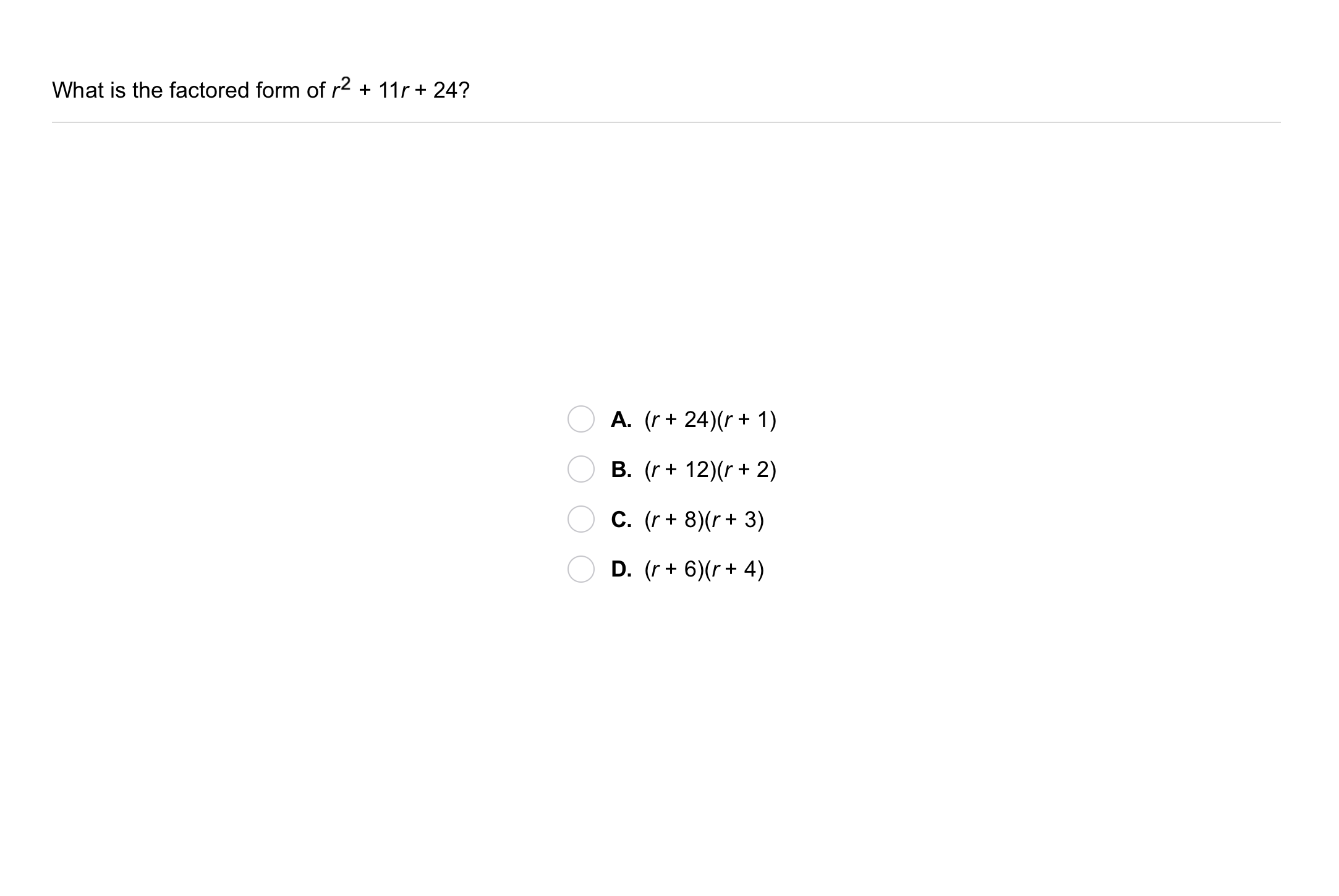2
2
10 pts
Problem 1 Got It?
A
B
C
D
3
10 pts
Problem 2 Got It? What is the factored form of the expression?

4
10 pts
Problem 2 Got It? Reasoning: Can you factor the expression? Explain.5
5
10 pts
Problem 3 Got It?
A
B
C
D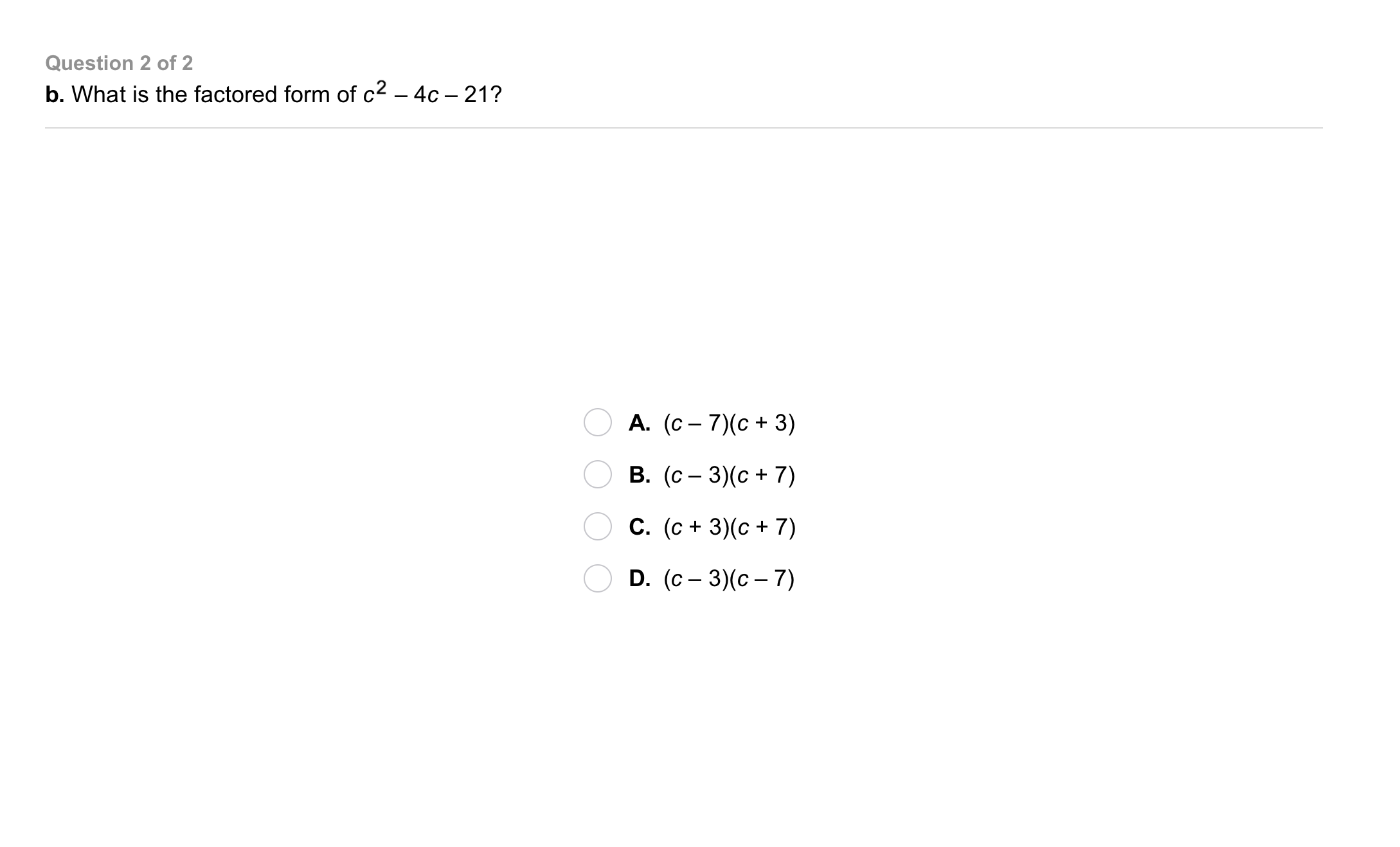6
6
10 pts
Problem 3 Got It?
A
B
C
D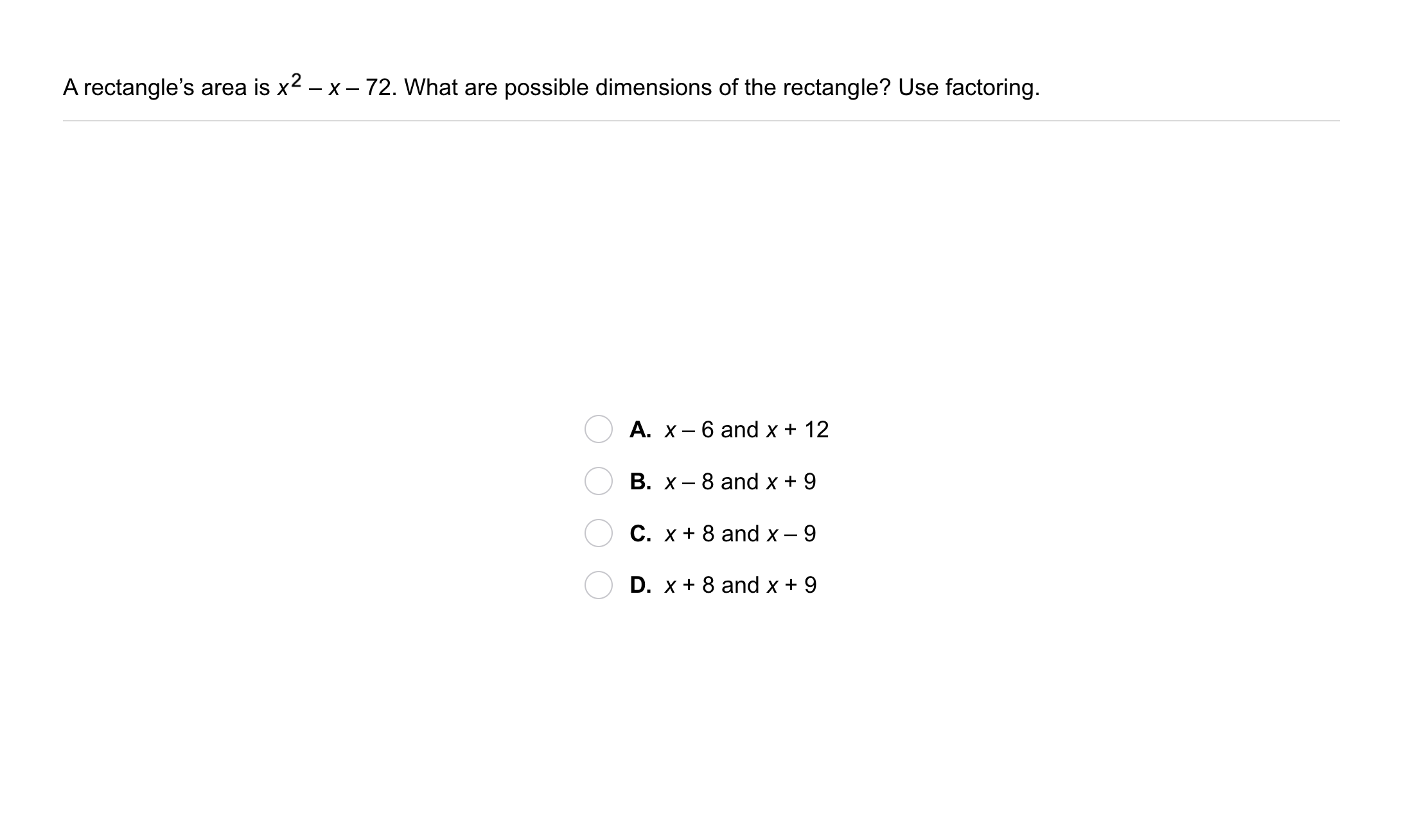7
7
10 pts
Problem 4 Got It?
A
B
C
D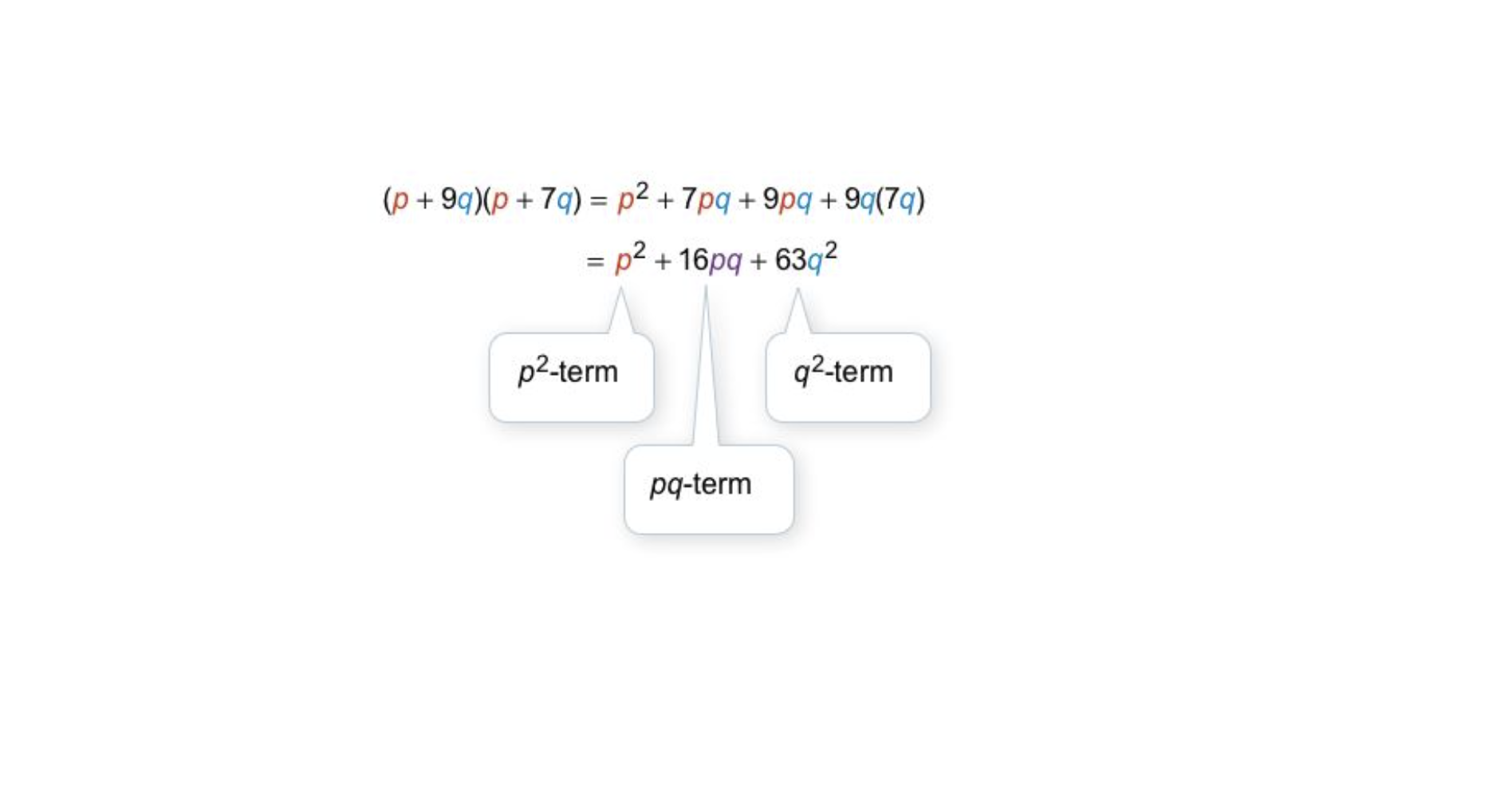8
8
10 pts
Problem 5 Got It?
A
B
C
D9
9
10 pts
A
B
C
D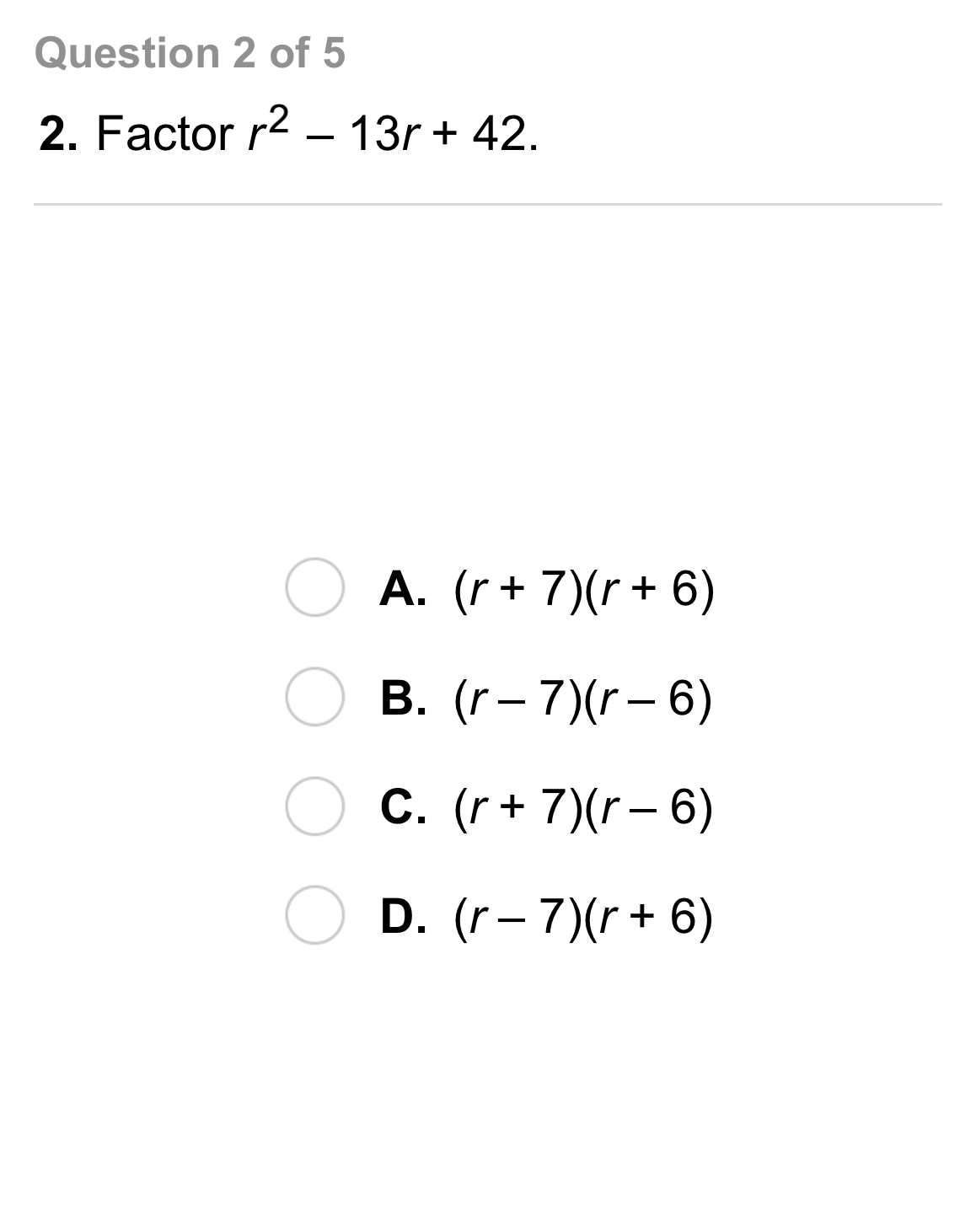10
10
10 pts
A
B
C
D11
11
10 pts
A
B
C
D12
12
10 pts
A
B
C
D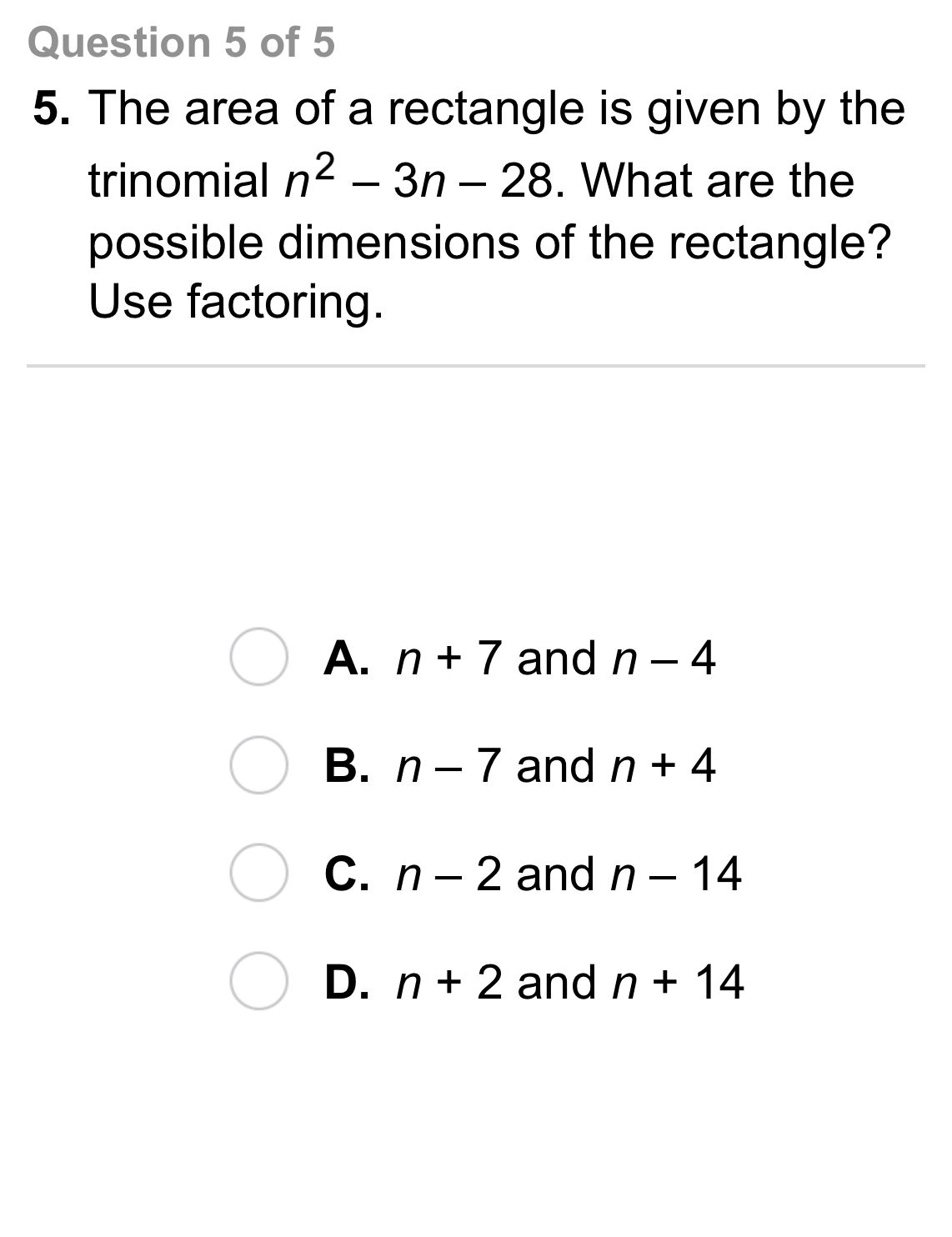13
13
10 pts
A
B
C
D14
5 pts
Analysis: Tell whether the sum of the factors of the constant terms should be positive or negative when you factor the trinomail.

positive
negative
15
5 pts
Analysis: Tell whether the sum of the factors of the constant terms should be positive or negative when you factor the trinomail.

positive
negative
16
5 pts
Analysis: Tell whether the sum of the factors of the constant terms should be positive or negative when you factor the trinomail.

positive
negative18
10 pts
Review Lesson 8-4: Simplify the product.

19
10 pts
Review Lesson 8-4: Simplify the product.

20
10 pts
Review Lesson 8-4: Simplify the product.

21
10 pts
Review Lesson 2-5: Solve each equation for x.

• x = b/a + d
• x = ad - b
• x = ad ÷ b
22
10 pts
Review Lesson 8-2: What is the GCF of the polynomial? Enter only a monomial.

23
10 pts
Review Lesson 8-2: What is the GCF of the polynomial? Enter only a monomial.

24
10 pts
Review Lesson 8-2: What is the GCF of the polynomial? Enter only a monomial.25
10 pts
Vocabulary Review: Use the Distributive Property. Match equivalent expressions.
• y(y + 2)
• (2y - 1)(y + 2)
• y(y² + 3y - 6)
• -1(y + 2)
• y³ + 3y² - 6y
• -y - 2
• y² + 2y
• 2y(y + 2) - 1(y + 2)26
10 pts
Use Your Vocabulary: Identify the trinomials.
• - 2h + 4
• + 3rs - 24
• -
• + g + 1
• -
• + 7x²y + 5xy² +
• Trinomials
27
100 pts
Notes: Take a clear picture or screenshot of your Cornell notes for this lesson. Upload it to the canvas. Zoom and pan as needed.

For a refresher on the Cornell note-taking system, click here.
28
10 pts
Reflection: Math Success
Add to my formatives list

Formative uses cookies to allow us to better understand how the site is used. By continuing to use this site, you consent to the Terms of Service and Privacy Policy.